# Factors and Multiples:

## 1. Factors:

### Factors of 4: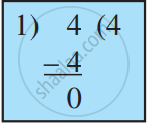Quotient is 4 Remainder is 0 4 = 1 × 4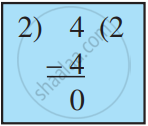Quotient is 2 Remainder is 0 4 = 2 × 2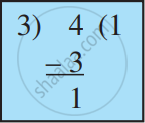Quotient is 1 Remainder is 1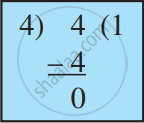Quotient is 1 Remainder is 0 4 = 4 × 1

We find that the number 4 can be written as: 4 = 1 × 4; 4 = 2 × 2;4 = 4 × 1 and knows that the numbers 1, 2, and 4 are exact divisors of 4. These numbers are called factors of 4.
A factor of a number is an exact divisor of that number.

### Multiples of 3:

A number is a multiple of each of its factors.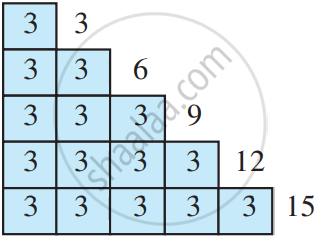Hence, We say that the numbers 3, 6, 9, 12, 15 are multiples of 3.
The list of multiples of 3 can be continued as 18, 21, 24,...
The list is endless.
Each of these multiples is greater than or equal to 3.

#### Facts about factors and multiples:

• 1 is a factor of every number.

• Every number is a factor of itself.

• Every factor of a number is an exact divisor of that number.

• Every factor is less than or equal to the given number.

• The number of factors of a given number are finite.

• Every multiple of a number is greater than or equal to that number.

• The number of multiples of a given number is infinite.

• Every number is a multiple of itself.

If you would like to contribute notes or other learning material, please submit them using the button below.

### Shaalaa.com

Factors and Multiples part-1 [00:14:51]
S
0%